Samacheer Kalvi 12th Business Maths Solutions Chapter 5 Numerical Methods Ex 5.2

Students can download 12th Business Maths Chapter 5 Numerical Methods Ex 5.2 Questions and Answers, Samacheer Kalvi 12th Business Maths Book Solutions Guide Pdf helps you to revise the complete Tamilnadu State Board New Syllabus and score more marks in your examinations.

Tamilnadu Samacheer Kalvi 12th Business Maths Solutions Chapter 5 Numerical Methods Ex 5.2

Question 1.
Using graphic method, find the value of y when x = 48 from the following data: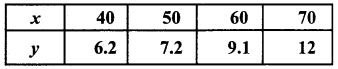Solution:
The given points are (40, 6.2), (50, 7.2) (60, 9.1) and (70, 12).
We plot the points on a graph with suitable scale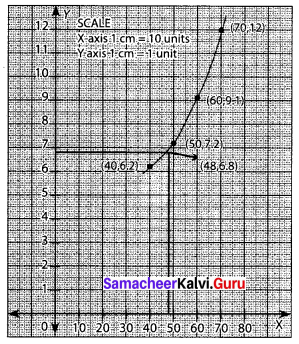The value of y when x = 48 is 6.8

Question 2.
The following data relates to indirect labour expenses and the level of output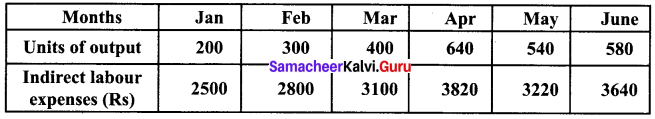Estimate the expenses at a level of output of 350 units, by using the graphic method.
Solution:
Take the units of output along the x-axis, labour expenses along the y-axis.
The points to be plotted are (200, 2500), (300, 2800) (400, 3100), (640, 3820), (540, 3220), (580, 3640)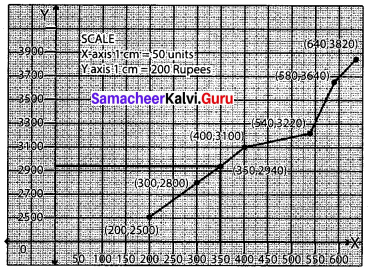From the graph, the expenses at a level of output of 350 units are ₹ 2940.Question 3.
Using Newton’s forward interpolation formula find the cubic polynomial.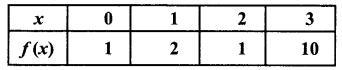Solution:
Newton’s forward interpolation formula is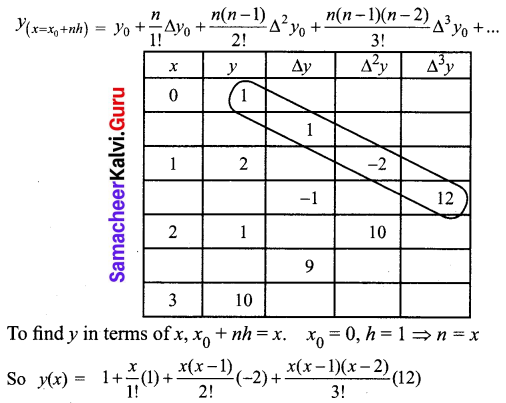y(x) = 1 + x – (x2 – x) + 2[x3 – 3x2 + 2x]
y(x) = 1 + x – x2 + x + 2x3 – 6x2 + 4x
f(x) = y = 2x3 – 7x2 + 6x + 1 is the required cubic polynomial

Question 4.
The population of a city in a census taken once in 10 years is given below.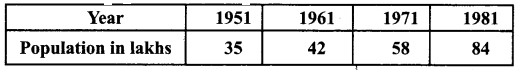Estimate the population in the year 1955.
Solution: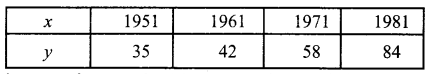Let ‘x’ denote the year of the census. Let ‘y’ represent the population in lakhs. We have to find the population in the year 1955 (i.e) the value of y when x = 1955. Since the value of y is required near the beginning of the table, we use Newton’s forward interpolation formula.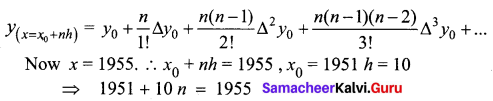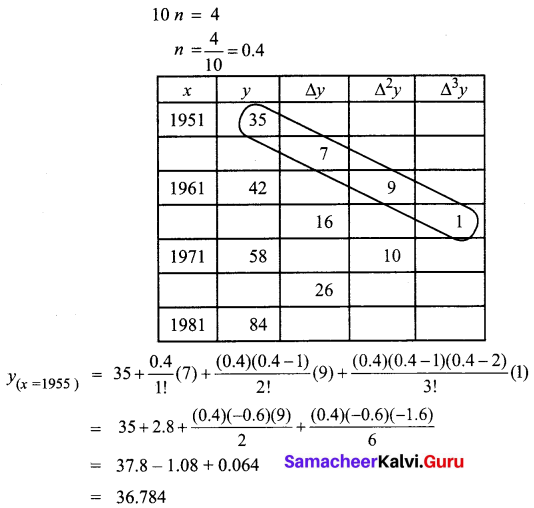Thus the estimated population in the year 1955 is 36.784 lakhsQuestion 5.
In an examination the number of candidates who secured marks between certain intervals was as follows: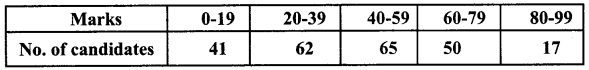Estimate the number of candidates whose marks are less than 70.
Solution:
Let x be the marks and y be the number of candidates. The given class intervals are not continuous. So we make them continuous and find the cumulative frequency.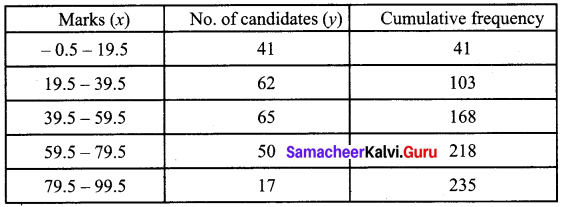The difference table is as follows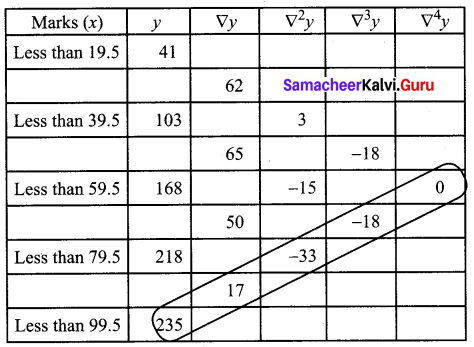Since the required value of y is near the end of the table, we use Newton’s backward interpolation formula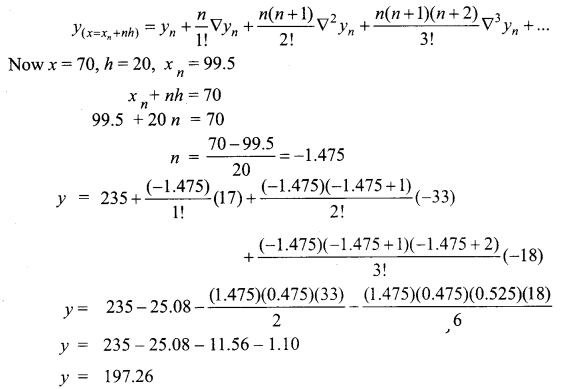Hence the estimated value of the number of candidates whose marks are less than 70 is 197

Question 6.
Find the value of f(x) when x = 32 from the following table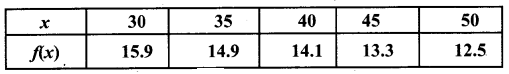Solution:
To find y = f(x) when x = 32 from the given table. Since the required value of y is near the beginning of the table, we use Newton’s forward interpolation formula.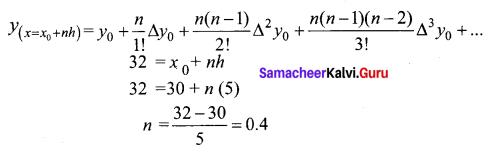The difference table is as follows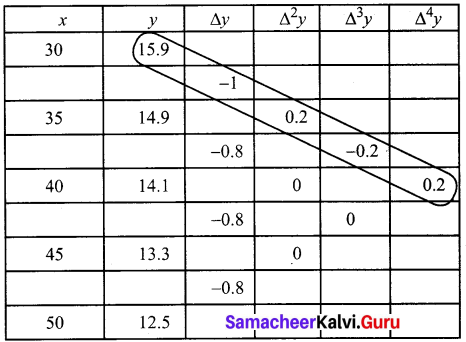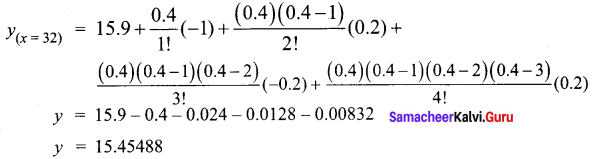Hence the value of f(x) when x = 32 is 15.45Question 7.
The following data gives the melting point of an alloy of lead and zinc where ‘t’ is the temperature in degree c and P is the percentage of lead in the alloy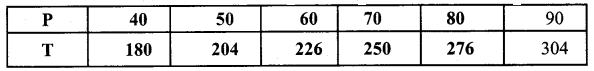Find the melting point of the alloy containing 84 per cent lead.
Solution:
To find T when P = 84. The required value of T is near the end of the table. So we use Newton’s backward interpolation formula.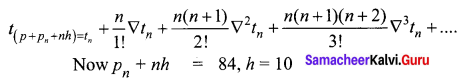⇒ 90 + n(10) = 84
⇒ n = $$\frac{84-90}{10}=\frac{-6}{10}=-0.6$$
The difference table is given below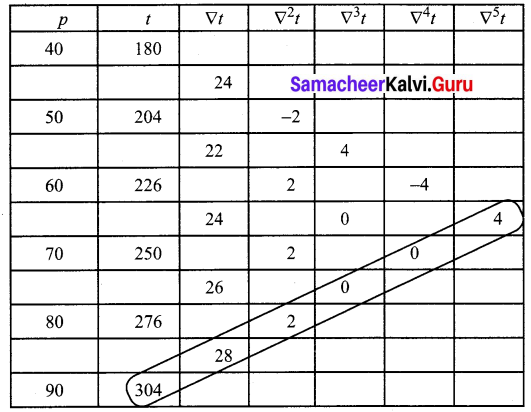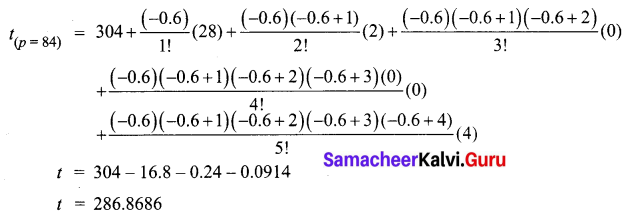Hence the melting point of the alloy containing 84 per cent lead is 286.9°C

Question 8.
Find f(2.8) from the following table.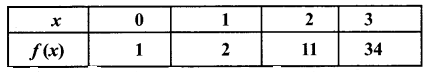Solution:
To find y = f(x) at x = 2.8 from the given table. We use Newton’s backward interpolation formula since the required value is near the end of the table.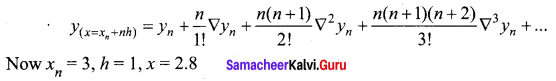2.8 = 3 + n(1)
n = 2.8 – 3 = -0.2
The difference table given below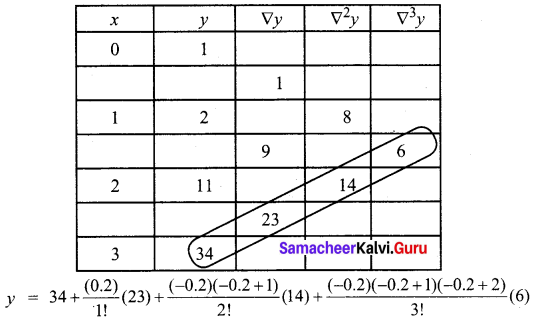y = 34 – 4.6 – 1.12 – 0.288
y = 27.992
Hence the value of f(x) at x = 2.8 is 27.992Question 9.
Using interpolation estimate the output of a factory in 1986 from the following data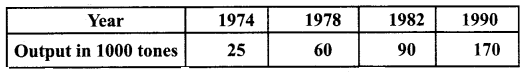Solution:
Let x denote the year and y represent the output. The x values are not equidistant. So we use Lagrange’s formula
x0 = 1974, y0 = 25
x1 = 1978, y1 = 60
x2 = 1982, y2 = 80
x3 = 1990, y3 = 170
For x = 1986 we have to find y value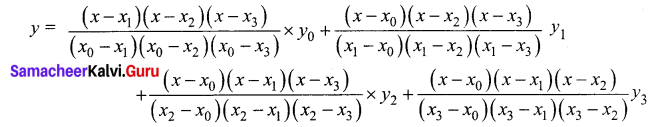We find the different values separately and substitute in the formula.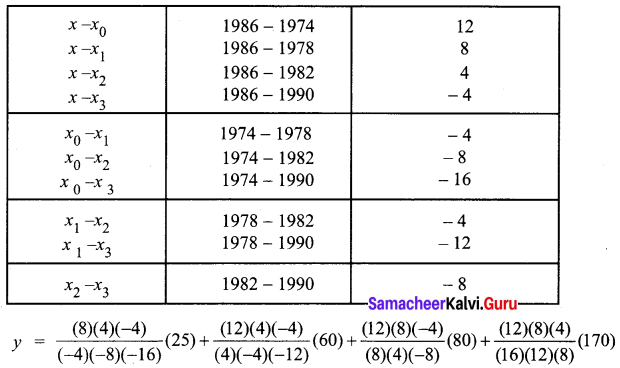y = 6.25 – 60 + 120 + 42.5
y = 108.75
The output of the factory in 1986 is 109 (thousand tonnes)

Question 10.
Use Lagrange’s formula and estimate from the following data the number of workers getting income not exceeding Rs. 26 per month.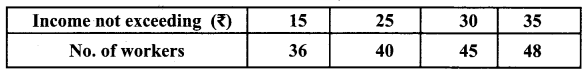Solution:
Let x represent the income per month and y denote the number of workers.
From the given data,
x0 = 15, y0 = 36
x1 = 25, y1 = 40
x2 = 30, y2 = 45
x3 = 35, y3 = 48
We have to find the value of y at x = 26
By Lagrange’s interpolation formula,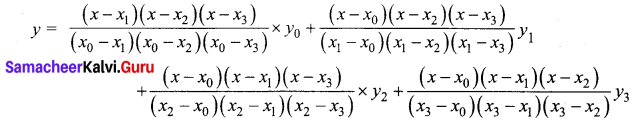The different values are given in the table below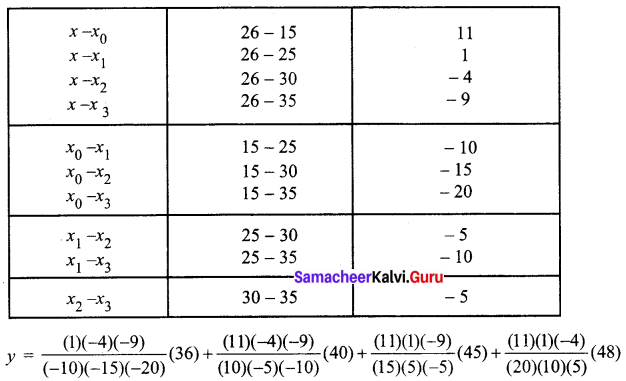y = -0.432 + 31.68 + 11.88 – 2.112
y = 41.016
Thus the number of workers getting income not exceeding Rs.26 per month is 42Question 11.
Using interpolation estimate the business done in 1985 from the following data.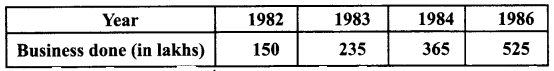Solution:
Let x denote the year of business and y (in lakhs) denote the amount of business.
From the given data,
x0 = 1982, y0 = 150
x1 = 1983, y1 = 235
x2 = 1984, y2 = 365
x3 = 1986, y3 = 525
We have to find the value of y when x = 1985.
By Lagrange’s interpolation formula,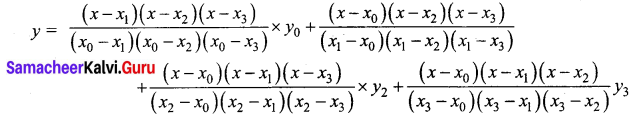We form a table for the different values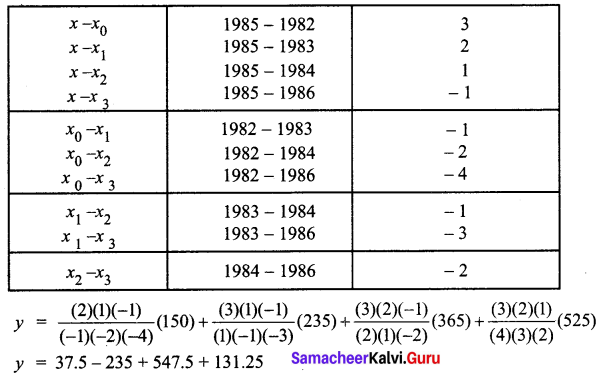y = 481.25
Thus the business done in the year 1985 is estimated as 481.25 lakhs

Question 12.
Using interpolation, find the value of f(x) when x = 15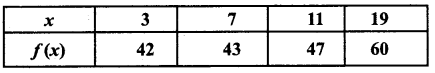Solution:
We have to find the value of y when x = 15.
From the given data,
x0 = 3, y0 = 42
x1 = 7, y1 = 43
x2 = 11, y2 = 47
x3 = 19, y3 = 60
Since the intervals are unequal, we use the Lagrange’s interpolation formula,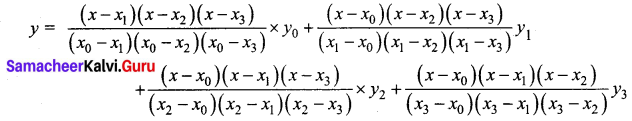The different values are given in the table below.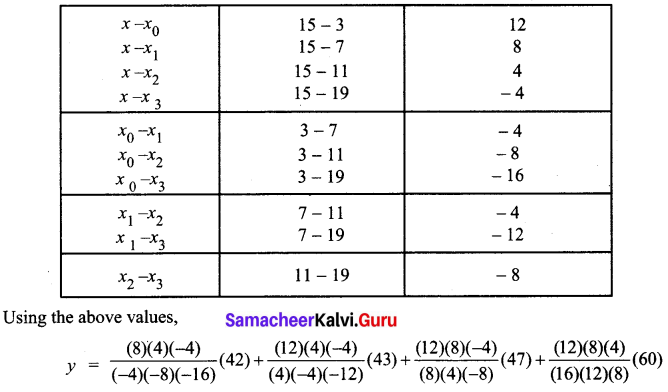y = 10.5 – 43 + 70.5 + 15
y = 53
Hence the value of f(x) when x = 15 is 53